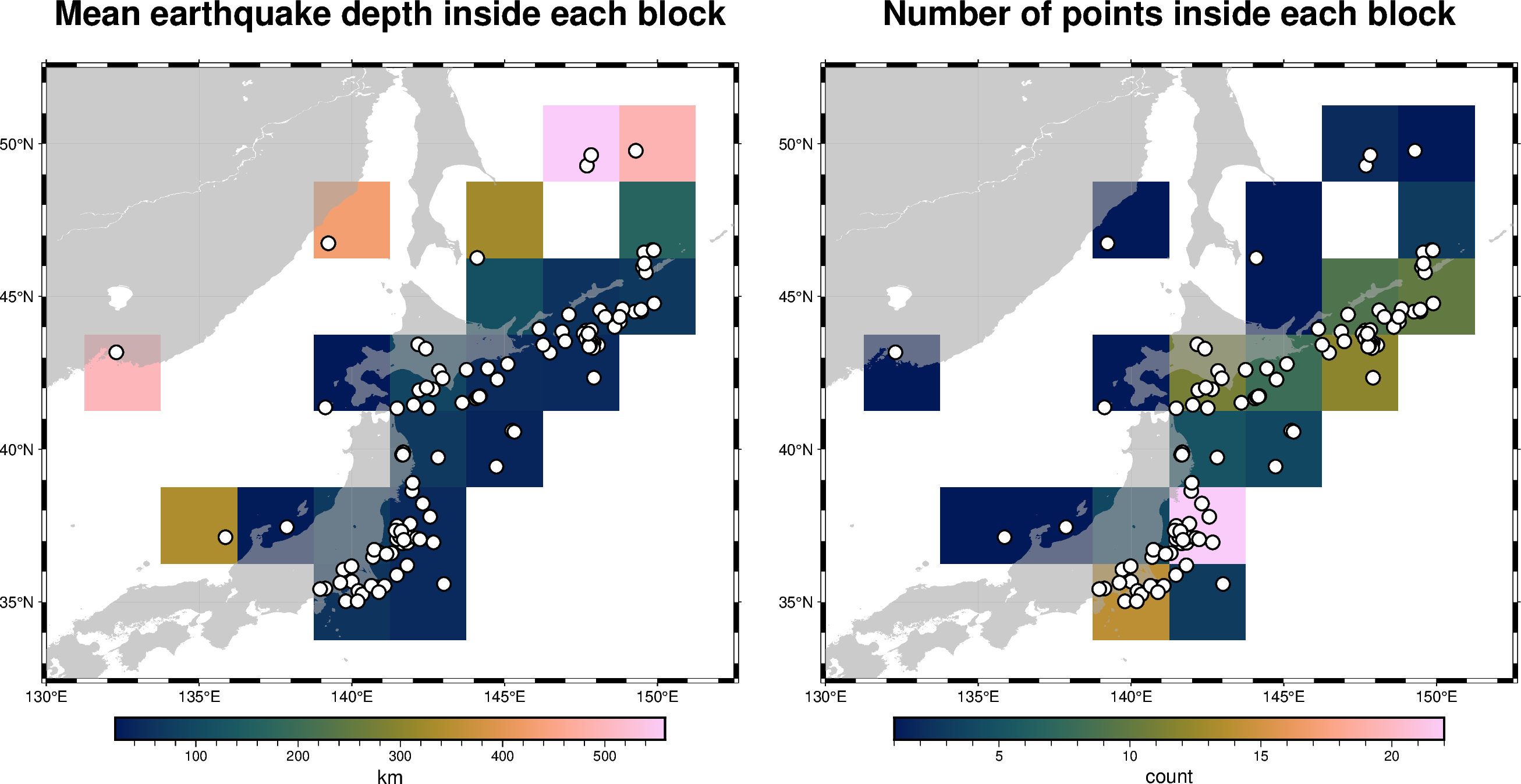# Blockmean

The `pygmt.blockmean` function calculates different quantities inside blocks/bins whose dimensions are defined via the `spacing` parameter. The following examples show how to calculate the averages of the given values inside each bin and how to report the number of points inside each bin.```import pygmt

# Select only needed columns
data = data[["longitude", "latitude", "depth_km"]]

# Set the region for the plot
region = [130, 152.5, 32.5, 52.5]
# Define spacing in x and y direction (150x150 arc-minute blocks)
spacing = "150m"

fig = pygmt.Figure()

# Calculate mean depth in kilometers from all events within
# 150x150 arc-minute bins using blockmean
df = pygmt.blockmean(data=data, region=region, spacing=spacing)
# Convert to grid
grd = pygmt.xyz2grd(data=df, region=region, spacing=spacing)

fig.grdimage(
grid=grd,
region=region,
frame=["af", "+tMean earthquake depth inside each block"],
cmap="batlow",
)
# Plot slightly transparent landmasses on top
fig.coast(land="darkgray", transparency=40)
# Plot original data points
fig.plot(x=data.longitude, y=data.latitude, style="c0.3c", fill="white", pen="1p,black")
fig.colorbar(frame=["x+lkm"])

fig.shift_origin(xshift="w+5c")

# Calculate number of total locations within 150x150 arc-minute bins
# with blockmean's summary parameter
df = pygmt.blockmean(data=data, region=region, spacing=spacing, summary="n")
grd = pygmt.xyz2grd(data=df, region=region, spacing=spacing)

fig.grdimage(
grid=grd,
region=region,
frame=["af", "+tNumber of points inside each block"],
cmap="batlow",
)
fig.coast(land="darkgray", transparency=40)
fig.plot(x=data.longitude, y=data.latitude, style="c0.3c", fill="white", pen="1p,black")
fig.colorbar(frame=["x+lcount"])

fig.show()
```

Total running time of the script: ( 0 minutes 2.160 seconds)

Gallery generated by Sphinx-Gallery